Updating search results...

# 21 Results

View
Selected filters:
• NC.M3.G-GPE.1Read the Fine Print
Rating
0.0 stars
Overview:

In this lesson, students explore the mathematics of architecture by investigating the unusual shapes of some of the rooms in the White House, such as the Blue Room's elliptical shape. They use a graph to plot, draw and determine the equation of an ellipse.

Subject:
Mathematics
Material Type:
Activity/Lab
Lesson Plan
Provider:
PBS
Author:
PBS Teachers Mathline
Date Added:
02/26/2019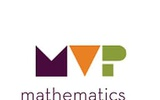Conditional Remix & Share Permitted
CC BY-NC-SA
Rating
0.0 stars
Overview:

The purpose of this task is for students to practice using the equation of the circle in different ways. In each case, they must draw inferences from the information given and use the information to find the equation of the circle or to justify conclusions about the circle. They will use the distance formula to find the measure of the radius and the midpoint formula to find the center of a circle.

Subject:
Math 3
Material Type:
Lesson
Author:
The Mathematics Vision Project
Date Added:
03/12/2020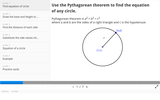Unrestricted Use
CC BY
Rating
2.0 stars
Overview:

Slides showing steps to derive the equation of a circle, using the Pythagorean Theorem

Subject:
Geometry
Material Type:
Interactive
Provider:
Michigan Virtual
Date Added:
07/19/2019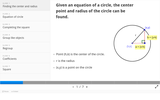Unrestricted Use
CC BY
Rating
1.0 stars
Overview:

Slides showing steps to solve for center and radius of a circle given an equation of a circle by completing the square.

Subject:
Geometry
Material Type:
Interactive
Provider:
Michigan Virtual
Date Added:
07/19/2019Conditional Remix & Share Permitted
CC BY-NC-SA
Rating
0.0 stars
Overview:

This purpose of this task is for students to connect their geometric understanding of circles as the set of all points equidistant from a center to the equation of a circle. In the task, students construct a circle using right triangles with a radius of 6 inches. This construction is intended to focus students on the Pythagorean Theorem and to use it to generate the equation of a circle centered at the origin. After constructing a circle at the origin, students are asked to use their knowledge of translations to consider how the equation would change if the center of the circle is translated.

Subject:
Math 3
Material Type:
Lesson
Author:
The Mathematics Vision Project
Date Added:
03/12/2020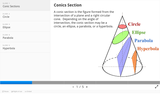Unrestricted Use
CC BY
Rating
2.33333333333 stars
Overview:

Image showing four conic sections, with an example of each type of equation; circle, ellipse, parabola, and hyperbola.

Subject:
Geometry
Material Type:
Interactive
Provider:
Michigan Virtual
Date Added:
07/19/2019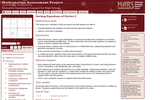Only Sharing Permitted
CC BY-NC-ND
Rating
4.0 stars
Overview:

This lesson unit is intended to help teachers assess how well students are able to: translate between the equations of circles and their geometric features; and sketch a circle from its equation.

Subject:
Geometry
Material Type:
Assessment
Lesson Plan
Provider:
Shell Center for Mathematical Education
Provider Set:
Mathematics Assessment Project (MAP)
Date Added:
07/31/2019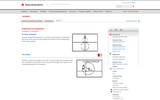Read the Fine Print
Rating
0.0 stars
Overview:

Students will discover the equation of circles of the form (x â€“ h)^2+ (y â€“ k)^2= r^2, where (h, k) is the center of the circle and r is the radius of the circle.

Subject:
Mathematics
Material Type:
Activity/Lab
Provider:
Texas Instruments
Date Added:
03/07/2018Read the Fine Print
Rating
0.0 stars
Overview:

In this Khan Academy activity, students will determine what the center of a circle is and its radius from a given graph.

Subject:
Mathematics
Material Type:
Activity/Lab
Provider:
Khan Academy
Date Added:
08/23/2018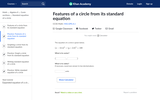Read the Fine Print
Rating
0.0 stars
Overview:

In this Khan Academy activity, students will determine what the center of a circle is and its radius from a given standard equation.

Subject:
Mathematics
Material Type:
Activity/Lab
Provider:
Khan Academy
Date Added:
08/23/2018Conditional Remix & Share Permitted
CC BY-NC-SA
Rating
0.0 stars
Overview:

Students write the equation for a circle in center-radius form, (x - a)2 (y - b)2 = r2 using the Pythagorean theorem or the distance formula.
Students write the equation of a circle given the center and radius. Students identify the center and radius of a circle given the equation.

Subject:
Math 3
Material Type:
Lesson
Author:
EngageNY
Date Added:
02/22/2020Conditional Remix & Share Permitted
CC BY-NC-SA
Rating
0.0 stars
Overview:

Students complete the square in order to write the equation of a circle in center-radius form.
Students recognize when a quadratic in x and y is the equation for a circle.

Subject:
Math 3
Material Type:
Lesson
Author:
EngageNY
Date Added:
02/22/2020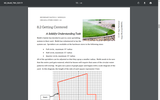Conditional Remix & Share Permitted
CC BY-NC-SA
Rating
0.0 stars
Overview:

The purpose of this task is to solidify understanding of the equation of the circle. The task begins with sketching circles and writing their equations. Students are challenged to reverse the process to find the center of the circle.

Subject:
Math 3
Material Type:
Lesson
Author:
The Mathematics Vision Project
Date Added:
03/12/2020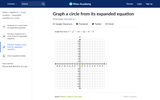Read the Fine Print
Rating
0.0 stars
Overview:

In this Khan Academy activity, students will graph a circle from its expanded equation.

Subject:
Mathematics
Material Type:
Activity/Lab
Provider:
Khan Academy
Date Added:
08/23/2018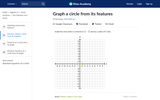Read the Fine Print
Rating
0.0 stars
Overview:

In this Khan Academy activity, students will graph a circle from its features.

Subject:
Mathematics
Material Type:
Activity/Lab
Provider:
Khan Academy
Date Added:
08/23/2018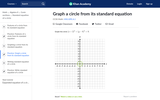Read the Fine Print
Rating
0.0 stars
Overview:

In this Khan Academy activity, students will graph a circle from its standard equation.

Subject:
Mathematics
Material Type:
Activity/Lab
Provider:
Khan Academy
Date Added:
08/23/2018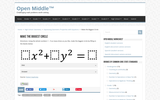Read the Fine Print
Rating
0.0 stars
Overview:

Using the whole numbers 1-9 as many times as you like, make the biggest circle by filling in the blanks

Subject:
Mathematics
Material Type:
Activity/Lab
Provider:
Open Middle
Date Added:
02/21/2018Read the Fine Print
Rating
0.0 stars
Overview:

This lesson unit is intended to help you assess how well students are able to: Use the Pythagorean theorem to derive the equation of a circle.
Translate between the geometric features of circles and their equations.

Subject:
Mathematics
Material Type:
Activity/Lab
Provider:
MARS, Mathematics Assessment Resource Service
Date Added:
11/15/2017Unrestricted Use
CC BY
Rating
0.0 stars
Overview:

Objectives • Students will develop the equation for a circle centered at (0, 0) from the Pythagorean Theorem.• Students will write equations of circles given a radius and center at (0, 0)• Students will solve equations of circles for y in terms of x.Vocabulary: • Radius • Center • Pythagorean TheoremAbout the Lesson • In this activity students will explore the creation of a circle skirt• Students will need familiarity with Pythagorean Theorem.• Students will need to be able to solve equations involving squares and square roots.

Subject:
Mathematics
Material Type:
Lesson Plan
Author:
JENNIFER BRINKLEY
Date Added:
08/14/2019Read the Fine Print
Rating
0.0 stars
Overview:

In this Khan Academy activity, students will write the equation of a circle in standard form.

Subject:
Mathematics
Material Type:
Activity/Lab
Provider:
Khan Academy
Date Added:
08/23/2018